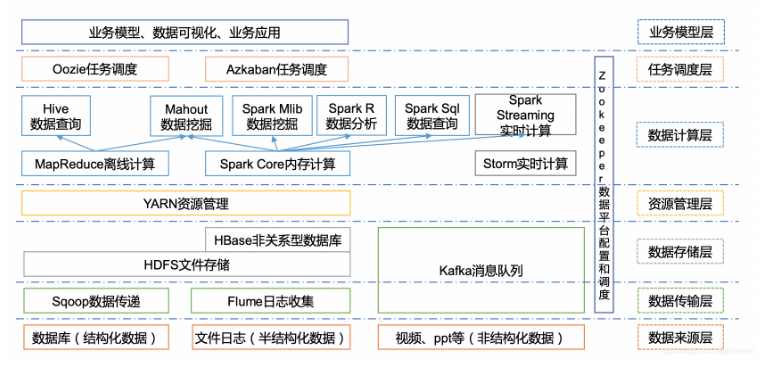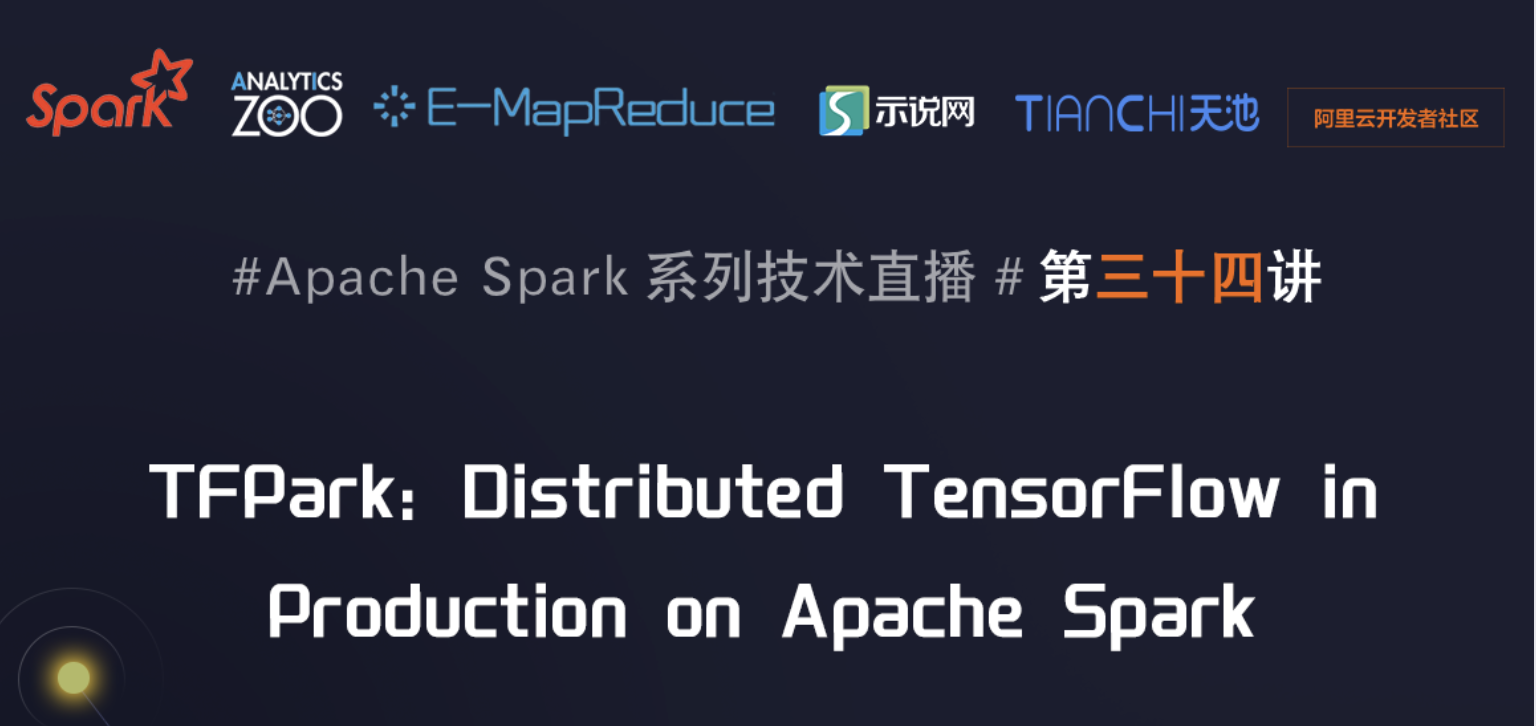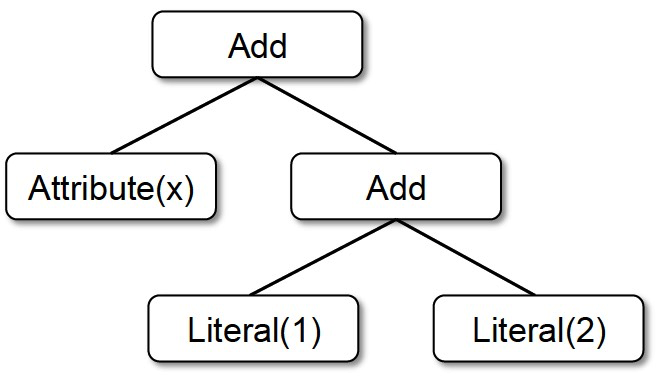# pyspark.mllib.feature module

+关注继续查看
Feature Extraction
Feature Extraction converts vague features in the raw data into concrete numbers for further analysis. In this section, we introduce two feature extraction technologies: TF-IDF and Word2Vec.
TF-IDF
Term frequency-inverse document frequency (TF-IDF) reflects the importance of a term (word) to the document in corpus. Denote a term by  , a document by , and the corpus by . Term frequency  is the number of times that term  appears in  while document frequency is the number of documents that contain the term.
If we only use term frequency to measure the importance, it is very easy to over-emphasize terms that appear very often but carry little information about the document, e.g., 'a', 'the', and 'of'. If a term appears very often across the corpus, it means it does not carry special information about a particular document. Inverse document frequency is a numerical measure of how much information a term provides:
where  is the total number of documents in the corpus. A smoothing term is applied to avoid dividing by zero for terms outside the corpus.
The TF-IDF measure is simply the product of TF and IDF:

pyspark.mllib.feature module

class pyspark.mllib.feature.HashingTF

Bases: object

Maps a sequence of terms to their term frequencies using hashing algorithm.
Method:

indexOf(term)

Returns the index of the input term.

transform(document)

Transforms the input document (list of terms) to term frequency vectors, or transform the RDD of document to RDD of term frequency vectors.

class pyspark.mllib.feature.IDFModel

Bases: pyspark.mllib.feature.JavaVectorTransformer

Represents an IDF model that can transform term frequency vectors.
Method:

transform(dataset)

Transforms term frequency (TF) vectors to TF-IDF vectors.

If minDocFreq was set for the IDF calculation, the terms which occur in fewer than minDocFreq documents will have an entry of 0.

Parameters:

dataset an RDD of term frequency vectors

Returns:

an RDD of TF-IDF vectors

class pyspark.mllib.feature.IDF (minDocFreq=0)

Bases: object

Inverse document frequency (IDF).

The standard formulation is used: idf = log((m + 1) / (d(t) + 1)), where m is the total number of documents and d(t) is the number of documents that contain term t.

This implementation supports filtering out terms which do not appear in a minimum number of documents (controlled by the variable minDocFreq). For terms that are not in at least minDocFreq documents, the IDF is found as 0, resulting in TF-IDFs of 0.
Method:

fit(dataset)

Computes the inverse document frequency.

Parameters:

dataset an RDD of term frequency vectors
Sample Code:

from pyspark import SparkContext

from pyspark.mllib.feature import HashingTF

from pyspark.mllib.feature import IDF

sc = SparkContext()

# Load documents (one per line).

documents = sc.textFile("data/mllib/document").map(lambda line: line.split(" "))

#Computes TF

hashingTF = HashingTF()

tf = hashingTF.transform(documents)

#Computes tfidef

tf.cache()

idf = IDF().fit(tf)

tfidf = idf.transform(tf)

for r in tfidf.collect(): print r

Data in document:

1 1 1 1

1 2 2 2

Output:

(1048576, , [0.0])

# 1048576 and  are total numbers of hash bracket and the hash bracket for this element respectively

# 0.0 is the TFIDF for word '1' in document 1.

(1048576, [485808, 559923], [0.0, 1.21639532432])

# 0.0 and 1. 21639532432 is the TFIDF for word '1' and word '2' in document 2.
Word2Vec
Word2Vec converts each word in documents into a vector. This technology is useful in many natural language processing applications such as named entity recognition, disambiguation, parsing, tagging and machine translation.
Mllib uses skip-gram model that is able to convert word in similar contexts into vectors that are close in vector space. Given a large dataset, skip-gram model can predict synonyms of a word with very high accuracy.

pyspark.mllib.feature module

class pyspark.mllib.feature.Word2Vec

Bases: object

Word2Vec creates vector representation of words in a text corpus.

Word2Vec used skip-gram model to train the model.
Method:

fit(data)

Computes the vector representation of each word in vocabulary.

Parameters:

data training data. RDD of list of string

Returns:

Word2VecModel instance

setLearningRate(learningRate)

Sets initial learning rate (default: 0.025).

setNumIterations(numIterations)

Sets number of iterations (default: 1), which should be smaller than or equal to number of partitions.

setNumPartitions(numPartitions)

Sets number of partitions (default: 1). Use a small number for accuracy.

setSeed(seed)

Sets random seed.

setVectorSize(vectorSize)

Sets vector size (default: 100).

class pyspark.mllib.feature.Word2VecModel

Bases: pyspark.mllib.feature.JavaVectorTransformer

class for Word2Vec model
Method:

findSynonyms(word, num)

Find synonyms of a word

Note: local use only

Parameters:  word a word or a vector representation of word

num number of synonyms to find

Returns:     array of (word, cosineSimilarity)

transform(word)

Transforms a word to its vector representation

Note: local use only

Parameters:

word a word

Returns:

vector representation of word(s)
Sample Code:

from pyspark import SparkContext

from pyspark.mllib.feature import Word2Vec

#Pippa Passes

sentence = "The year is at the spring \

And the day is at the morn; \

Morning is at seven;  \

The hill-side is dew-pearled; \

The lark is on the wing; \

The snai is on the thorn; \

God's in His heaven; \

All's right with the world "

sc = SparkContext()

#Generate doc

localDoc = [sentence, sentence]

doc = sc.parallelize(localDoc).map(lambda line: line.split(" "))

#Convect word in doc to vectors.

model = Word2Vec().fit(doc)

#Print the vector of "The"

vec = model.transform("The")

print vec

#Find the synonyms of "The"

syms = model.findSynonyms("The", 5)

print [s for s in syms]

Output:

[-0.00352853513323,0.00335159664974,-0.00598029373214,0.00399478571489,-0.00198440207168,-0.00294396048412,-0.00279111019336,0.00574737275019,-0.00628866581246,-0.00110566907097,-0.00108648219611,-0.00195649731904,0.00195016933139,0.00108497566544,-0.00230407039635,0.00146713317372,0.00322529440746,-0.00460519595072,0.0029725972563,-0.0018835098017,-1.38119357871e-05,0.000757675385103,-0.00189483352005,-0.00201138551347,0.00030658338801,0.00328158447519,-0.00367985945195,0.003532753326,-0.0019905695226,0.00628945976496,-0.00582657754421,0.00338909355924,0.00336381071247,-0.00497342273593,0.000185315642739,0.00409715576097,0.00307129183784,-0.00160020322073,0.000823577167466,0.00359133118764,0.000429257488577,-0.00509830284864,0.00443912763149,0.00010487002146,0.00211782287806,0.00373624730855,0.00489703053609,-0.00397138809785,0.000249207223533,-0.00378827378154,-0.000930541602429,-0.00113072514068,-0.00480769388378,-0.00129892374389,-0.0016206469154,0.00158304872457,-0.00206038192846,-0.00416553160176,0.00646342104301,0.00531594920903,0.00196505431086,0.00229385774583,-0.00256532337517,1.66955578607e-05,-0.00372383627109,0.00685756560415,0.00612043589354,-0.000518668384757,0.000620941573288,0.00244942889549,-0.00180160428863,-0.00129932863638,-0.00452549103647,0.00417296867818,-0.000546502880752,-0.0016888830578,-0.000340467959177,-0.00224090646952,0.000401715224143,0.00230841850862,0.00308039737865,-0.00271077733487,-0.00409514643252,-0.000891392992344,0.00459721498191,0.00295961694792,0.00211095809937,0.00442661950365,-0.001312403474,0.00522524351254,0.00116976187564,0.00254187034443,0.00157006899826,-0.0026122755371,0.00510979117826,0.00422499561682,0.00410514092073,0.00415299832821,-0.00311993830837,-0.00247424701229]

[u'', u'the', u'\t', u'is', u'at'] #The synonyms of "The"

Data Transformation
Data Transformation manipulates values in each dimension of vectors according to a predefined rule. Vectors that have gone through transformation can be used for future processing.
We introduce two types of data transformation: StandardScaler and Normalizer in this section.
StandardScaler
StandardScaler makes vectors in the dataset have zero-mean (when subtracting the mean in the enumerator) and unit-variance.

pyspark.mllib.feature module

class pyspark.mllib.feature.StandardScalerModel

Bases: pyspark.mllib.feature.JavaVectorTransformer

Represents a StandardScaler model that can transform vectors.
Method:

transform(vector)

Applies standardization transformation on a vector.

Parameters:

vector Vector or RDD of Vector to be standardized.

Returns:

Standardized vector. If the variance of a column is zero, it will return default 0.0 for the column with zero variance.

class pyspark.mllib.feature.StandardScaler(withMean=False, withStd=True)

Bases: object

Standardizes features by removing the mean and scaling to unit variance using column summary statistics on the samples in the training set.

If withMean is true, all the dimension of each vector subtract the mean of this dimension.

If withStd is true, all the dimension of each vector divides the length of the vector.
Method:

fit(dataset)

Computes the mean and variance and stores as a model to be used for later scaling.

Parameters:

data The data used to compute the mean and variance to build the transformation model.

Returns:

a StandardScalarModel
Sample Code:

from pyspark.mllib.feature import Normalizer

from pyspark.mllib.linalg import Vectors

from pyspark import SparkContext

from pyspark.mllib.feature import StandardScaler

sc = SparkContext()

vs = [Vectors.dense([-2.0, 2.3, 0]), Vectors.dense([3.8, 0.0, 1.9])]

dataset = sc.parallelize(vs)

#all false, do nothing.

standardizer = StandardScaler(False, False)

model = standardizer.fit(dataset)

result = model.transform(dataset)

for r in result.collect(): print r

print("\n")

#deducts the mean

standardizer = StandardScaler(True, False)

model = standardizer.fit(dataset)

result = model.transform(dataset)

for r in result.collect(): print r

print("\n")

#divides the length of vector

standardizer = StandardScaler(False, True)

model = standardizer.fit(dataset)

result = model.transform(dataset)

for r in result.collect(): print r

print("\n")

#Deducts min first, divides the length of vector later

standardizer = StandardScaler(True, True)

model = standardizer.fit(dataset)

result = model.transform(dataset)

for r in result.collect(): print r

print("\n")
Output:

#all false, do nothing.

[-2.0,2.3,0.0]

[3.8,0.0,1.9]

#deducts the mean

[-2.9,1.15,-0.95]

[2.9,-1.15,0.95]

#divides the length of vector

[-0.487659849094,1.41421356237,0.0]

[0.926553713279,0.0,1.41421356237]

#Deducts min first, divides the length of vector later

[-0.707106781187,0.707106781187,-0.707106781187]

[0.707106781187,-0.707106781187,0.707106781187]
Normalizer
Normalizer scales vectors by divide each dimension of the vector with a Lp norm.
For 1 <= p <= infinite, Lp norm is calculated as follows: sum(abs(vector)p)(1/p).
For p = infinite, Lp norm is max(abs(vector))

pyspark.mllib.feature module

class pyspark.mllib.feature.Normalizer(p=2.0)

Bases: pyspark.mllib.feature.VectorTransformer
Method:

transform(vector)

Applies unit length normalization on a vector.

Parameters:

vector vector or RDD of vector to be normalized.

Returns:

normalized vector. If the norm of the input is zero, it will return the input vector.
Sample Code:

from pyspark.mllib.feature import Normalizer

from pyspark.mllib.linalg import Vectors

from pyspark import SparkContext

sc = SparkContext()

# v = [0.0, 1.0, 2.0]

v = Vectors.dense(range(3))

# p = 1

nor = Normalizer(1)

print (nor.transform(v))

# p = 2

nor = Normalizer(2)

print (nor.transform(v))

# p = inf

nor = Normalizer(p=float("inf"))

print (nor.transform(v))

Output:

[0.0, 0.3333333333, 0.666666667]

[0.0, 0.4472135955, 0.894427191]

[0.0, 0.5, 1.0]

##pyspark.mllib.feature module

class pyspark.mllib.feature.HashingTF

Bases: object

Maps a sequence of terms to their term frequencies using hashing algorithm.

## Method:

indexOf(term)

Returns the index of the input term.

transform(document)

Transforms the input document (list of terms) to term frequency vectors, or transform the RDD of document to RDD of term frequency vectors.

class pyspark.mllib.feature.IDFModel

Bases: pyspark.mllib.feature.JavaVectorTransformer

Represents an IDF model that can transform term frequency vectors.

## Method:

transform(dataset)

Transforms term frequency (TF) vectors to TF-IDF vectors.

If minDocFreq was set for the IDF calculation, the terms which occur in fewer than minDocFreq documents will have an entry of 0.

 Parameters: dataset an RDD of term frequency vectors Returns: an RDD of TF-IDF vectors

class pyspark.mllib.feature.IDF(minDocFreq=0)

Bases: object

Inverse document frequency (IDF).

The standard formulation is used: idf = log((m + 1) / (d(t) + 1)), where m is the total number of documents and d(t) is the number of documents that contain term t.

This implementation supports filtering out terms which do not appear in a minimum number of documents (controlled by the variable minDocFreq). For terms that are not in at least minDocFreq documents, the IDF is found as 0, resulting in TF-IDFs of 0.

## Method:

fit(dataset)

Computes the inverse document frequency.

 Parameters: dataset an RDD of term frequency vectors

## Sample Code:

from pyspark import SparkContext

from pyspark.mllib.feature import HashingTF

from pyspark.mllib.feature import IDF

sc = SparkContext()

# Load documents (one per line).

documents = sc.textFile("data/mllib/document").map(lambda line: line.split(" "))

#Computes TF

hashingTF = HashingTF()

tf = hashingTF.transform(documents)

#Computes tfidef

tf.cache()

idf = IDF().fit(tf)

tfidf = idf.transform(tf)

for r in tfidf.collect():print r

Data in document:

1111

1222

Output:

(1048576,,[0.0])

# 1048576 and  are total numbers of hash bracket and the hash bracket for this element respectively

# 0.0 is the TFIDF for word '1'in document 1.

(1048576,[485808,559923],[0.0,1.21639532432])

# 0.0 and 1.21639532432 is the TFIDF for word '1' and word '2' in document 2.

## Mllib uses skip-gram model that is able to convert word in similar contexts into vectors that are close in vector space. Given a large dataset, skip-gram model can predict synonyms of a word with very high accuracy.

pyspark.mllib.feature module

class pyspark.mllib.feature.Word2Vec

Bases: object

Word2Vec creates vector representation of words in a text corpus.

Word2Vec used skip-gram model to train the model.

## Method:

fit(data)

Computes the vector representation of each word in vocabulary.

 Parameters: data training data. RDD of list of string Returns: Word2VecModel instance

setLearningRate(learningRate)

Sets initial learning rate (default: 0.025).

setNumIterations(numIterations)

Sets number of iterations (default: 1), which should be smaller than or equal to number of partitions.

setNumPartitions(numPartitions)

Sets number of partitions (default: 1). Use a small number for accuracy.

setSeed(seed)

Sets random seed.

setVectorSize(vectorSize)

Sets vector size (default: 100).

class pyspark.mllib.feature.Word2VecModel

Bases: pyspark.mllib.feature.JavaVectorTransformer

class for Word2Vec model

## Method:

findSynonyms(wordnum)

Find synonyms of a word

Note: local use only

Parameters:  worda word or a vector representation of word

numnumber of synonyms to find

Returns:     array of (word, cosineSimilarity)

transform(word)

Transforms a word to its vector representation

Note: local use only

 Parameters: word a word Returns: vector representation of word(s)

## Sample Code:

from pyspark import SparkContext

from pyspark.mllib.feature import Word2Vec

#Pippa Passes

sentence ="The year is at the spring \

And the day is at the morn; \

Morning is at seven;  \

The hill-side is dew-pearled; \

The lark is on the wing; \

The snai is on the thorn; \

God's in His heaven; \

All's right with the world "

sc = SparkContext()

#Generate doc

localDoc =[sentence, sentence]

doc = sc.parallelize(localDoc).map(lambda line: line.split(" "))

#Convect word in doc to vectors.

model = Word2Vec().fit(doc)

#Print the vector of "The"

vec = model.transform("The")

print vec

#Find the synonyms of "The"

syms = model.findSynonyms("The",5)

print[sfor s in syms]

## Output:

[-0.00352853513323,0.00335159664974,-0.00598029373214,0.00399478571489,-0.00198440207168,-0.00294396048412,-0.00279111019336,0.00574737275019,-0.00628866581246,-0.00110566907097,-0.00108648219611,-0.00195649731904,0.00195016933139,0.00108497566544,-0.00230407039635,0.00146713317372,0.00322529440746,-0.00460519595072,0.0029725972563,-0.0018835098017,-1.38119357871e-05,0.000757675385103,-0.00189483352005,-0.00201138551347,0.00030658338801,0.00328158447519,-0.00367985945195,0.003532753326,-0.0019905695226,0.00628945976496,-0.00582657754421,0.00338909355924,0.00336381071247,-0.00497342273593,0.000185315642739,0.00409715576097,0.00307129183784,-0.00160020322073,0.000823577167466,0.00359133118764,0.000429257488577,-0.00509830284864,0.00443912763149,0.00010487002146,0.00211782287806,0.00373624730855,0.00489703053609,-0.00397138809785,0.000249207223533,-0.00378827378154,-0.000930541602429,-0.00113072514068,-0.00480769388378,-0.00129892374389,-0.0016206469154,0.00158304872457,-0.00206038192846,-0.00416553160176,0.00646342104301,0.00531594920903,0.00196505431086,0.00229385774583,-0.00256532337517,1.66955578607e-05,-0.00372383627109,0.00685756560415,0.00612043589354,-0.000518668384757,0.000620941573288,0.00244942889549,-0.00180160428863,-0.00129932863638,-0.00452549103647,0.00417296867818,-0.000546502880752,-0.0016888830578,-0.000340467959177,-0.00224090646952,0.000401715224143,0.00230841850862,0.00308039737865,-0.00271077733487,-0.00409514643252,-0.000891392992344,0.00459721498191,0.00295961694792,0.00211095809937,0.00442661950365,-0.001312403474,0.00522524351254,0.00116976187564,0.00254187034443,0.00157006899826,-0.0026122755371,0.00510979117826,0.00422499561682,0.00410514092073,0.00415299832821,-0.00311993830837,-0.00247424701229]

[u'',u'the',u'\t',u'is',u'at'#The synonyms of "The"

## StandardScaler makes vectors in the dataset have zero-mean (when subtracting the mean in the enumerator) and unit-variance.

pyspark.mllib.feature module

class pyspark.mllib.feature.StandardScalerModel

Bases: pyspark.mllib.feature.JavaVectorTransformer

Represents a StandardScaler model that can transform vectors.

## Method:

transform(vector)

Applies standardization transformation on a vector.

 Parameters: vector Vector or RDD of Vector to be standardized. Returns: Standardized vector. If the variance of a column is zero, it will return default 0.0 for the column with zero variance.

class pyspark.mllib.feature.StandardScaler(withMean=FalsewithStd=True)

Bases: object

Standardizes features by removing the mean and scaling to unit variance using column summary statistics on the samples in the training set.

If withMean is true, all the dimension of each vector subtract the mean of this dimension.

If withStd is true, all the dimension of each vector divides the length of the vector.

## Method:

fit(dataset)

Computes the mean and variance and stores as a model to be used for later scaling.

 Parameters: data The data used to compute the mean and variance to build the transformation model. Returns: a StandardScalarModel

## Sample Code:

from pyspark.mllib.feature import Normalizer

from pyspark.mllib.linalg import Vectors

from pyspark import SparkContext

from pyspark.mllib.feature import StandardScaler

sc = SparkContext()

vs =[Vectors.dense([-2.0,2.3,0]), Vectors.dense([3.8,0.0,1.9])]

dataset = sc.parallelize(vs)

#all false, do nothing.

standardizer = StandardScaler(False,False)

model = standardizer.fit(dataset)

result = model.transform(dataset)

for r in result.collect():print r

print("\n")

#deducts the mean

standardizer = StandardScaler(True,False)

model = standardizer.fit(dataset)

result = model.transform(dataset)

for r in result.collect():print r

print("\n")

#divides the length of vector

standardizer = StandardScaler(False,True)

model = standardizer.fit(dataset)

result = model.transform(dataset)

for r in result.collect():print r

print("\n")

#Deducts min first, divides the length of vector later

standardizer = StandardScaler(True,True)

model = standardizer.fit(dataset)

result = model.transform(dataset)

for r in result.collect():print r

print("\n")

## Output:

#all false, do nothing.

[-2.0,2.3,0.0]

[3.8,0.0,1.9]

#deducts the mean

[-2.9,1.15,-0.95]

[2.9,-1.15,0.95]

#divides the length of vector

[-0.487659849094,1.41421356237,0.0]

[0.926553713279,0.0,1.41421356237]

#Deducts min first, divides the length of vector later

[-0.707106781187,0.707106781187,-0.707106781187]

[0.707106781187,-0.707106781187,0.707106781187]

## For p = infinite, Lp norm is max(abs(vector))

pyspark.mllib.feature module

class pyspark.mllib.feature.Normalizer(p=2.0)

Bases: pyspark.mllib.feature.VectorTransformer

## Method:

transform(vector)

Applies unit length normalization on a vector.

 Parameters: vector vector or RDD of vector to be normalized. Returns: normalized vector. If the norm of the input is zero, it will return the input vector.

## Sample Code:

from pyspark.mllib.feature import Normalizer

from pyspark.mllib.linalg import Vectors

from pyspark import SparkContext

sc = SparkContext()

# v = [0.0, 1.0, 2.0]

= Vectors.dense(range(3))

# p = 1

nor = Normalizer(1)

print(nor.transform(v))

# p = 2

nor = Normalizer(2)

print(nor.transform(v))

# p = inf

nor = Normalizer(p=float("inf"))

print(nor.transform(v))

## Output:

[0.0,0.3333333333,0.666666667]

[0.0,0.4472135955,0.894427191]

[0.0,0.5,1.0]|
2月前
|

34 1
|
3月前
|
SQL 机器学习/深度学习 分布式计算
spark与pyspark教程（一）
spark与pyspark教程（一）
135 0
|
7月前
|

PySpark ML（转换器）
PySpark转换器详解
61 0
|

Spark 中 ML 和 MLlib 的特点和区别
Spark 中 ML 和 MLlib 的特点和区别
299 0
|

）Scala 是一门多范式（multi-paradigm）的编程语言，设计初衷是要集成面向对象编程和函数式编程的各种特性。 Scala 运行在 Java 虚拟机上，并兼容现有的 Java 程序。
241 0|

7月23日社区直播【TFPark: Distributed TensorFlow in Production on Apache Spark】
TFPark是开源AI平台Analytics Zoo中一个模块，它的可以很方便让用户在Spark集群中分布式地进行TensorFlow模型的训练和推断。一方面，TFPark利用Spark将TensorFlow 定义的AI训练或推理任务无缝的嵌入到用户的大数据流水线中，而无需对现有集群做任何修改；另一方面TFPark屏蔽了复杂的分布式系统逻辑，可以将单机开发的AI应用轻松扩展到几十甚至上百节点上。本次分享将介绍TFPark的使用，内部实现以及在生产环境中的实际案例。
579 0|

Spark Codegen浅析
Codegen是Spark Runtime优化性能的关键技术，核心在于动态生成java代码、即时compile和加载，把解释执行转化为编译执行。Spark Codegen分为Expression级别和WholeStage级别，分别针对表达式计算和全Stage计算做代码生成，都取得了数量级的性能提升。本文浅析Spark Codegen技术原理。
2199 0|
Apache 流计算 消息中间件
Streaming with Apache Training